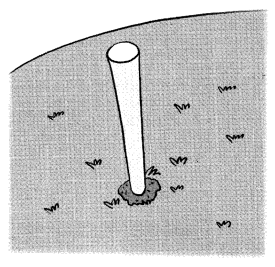# Math in Focus Grade 3 Chapter 2 Practice 5 Answer Key Using Front-End Estimation

Go through the Math in Focus Grade 3 Workbook Answer Key Chapter 2 Practice 5 Using Front-End Estimation to finish your assignments.

## Math in Focus Grade 3 Chapter 2 Practice 5 Answer Key Using Front-End Estimation

Question 1.
473 ___

Question 2.
801 ___

Question 3.
198 ____

Question 4.
5,147 ___

Question 5.
7,061 ___

Question 6.
9,625 ____

Find the sum. Use front-end estimation to check that each answer is reasonable.

Example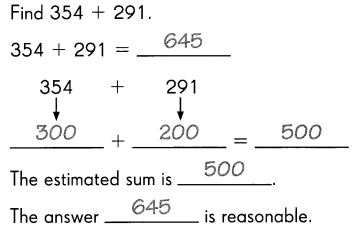Question 7.
Find 312 + 526.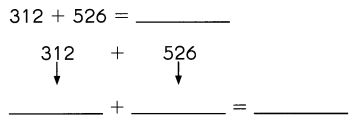The estimated sum is ___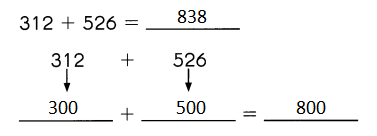The estimated sum is 800
The answer is 838 is reasonable

Find the sum. Use front-end estimation to check that each answer is reasonable.

Question 8.
Find 364 + 509.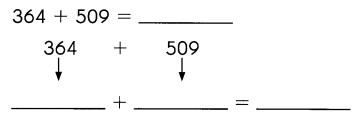The estimated sum is ___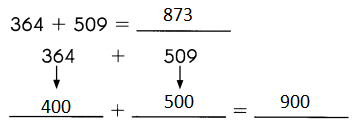The estimated sum is 900

Question 9.
Find 286 + 473.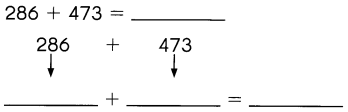The estimated sum is ___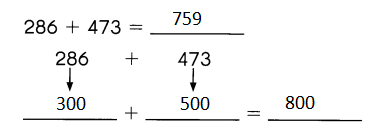The estimated sum is 800

Find the difference. Use front-end estimation to check that each answer is reasonable.

Example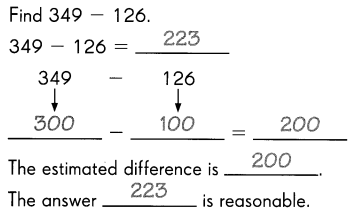Question 10.
Find 618 – 372.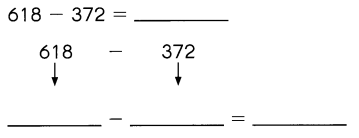The estimated difference is ___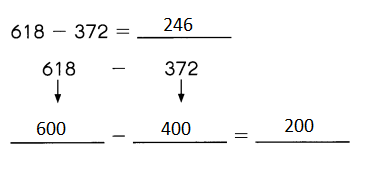The estimated difference is 200

Question 11.
Find 936 – 528.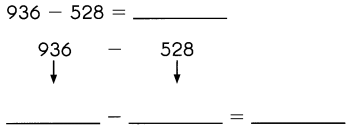The estimated difference is ___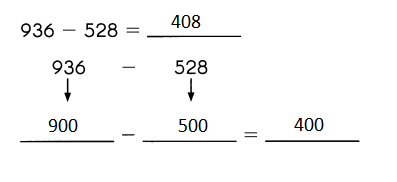The estimated difference is 400

Question 12.
Find 759 – 236.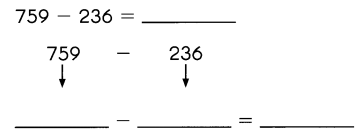The estimated difference is ___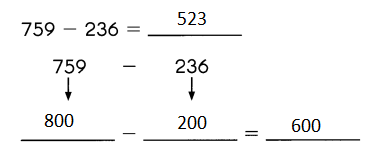The estimated difference is 600
The answer 523 is not reasonable.

Solve.

Question 13.
The length of a train engine is 439 centimeters. The length of the carriage is about 558 centimeters. Estimate the total length of the train and the carriage.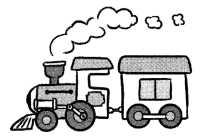Given,
The length of a train engine is 439 centimeters. The length of the carriage is about 558 centimeters.
439 is near to 400 and 558 is near to 600.
So, 400 + 600 = 1000
Therefore, the Estimation of the total length of the train and the carriage is 1000 centimeters.

Question 14.
A wooden pole is 356 centimeters long. 104 centimeters of it is driven into the ground. About what height of the wooden pole is above the ground?